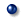Flash Physics animation

When the name below is clicked, the animation made by 'FLASH' is displayed.
Please click "Return" of a browser when it returns to this menu panel.v-t graph of speed movement(One second) 　　　 　The area of the v-t graph in the speed movement shows the distance.Displacement 　　　 　Displacement shows how into which direction the position changed from the starting point by a straight line distance.Synthetic speed 　　　　 　When the boat advances on the river that moves like the belt conveyer, the speed of the river is added.Relative speed 　　　 　In the position of the object seen from the observer who is moving, it is how much relative speed in which direction each unit time whether the distance parts.Acceleration 　　　 　One arrow red shows the acceleration. 　A yellow arrow into which the below changes shows the speed at the time.Monkey hunting 　　　　 　It collides whenever the object that falls freely and the object that projects orthorhombic that aims at it are launched at the same time.v-t graph of uniformly accelerated motion 　　　 　The area of the v-t graph in the uniformly accelerated motion shows the distance.v-t graph of uniformly accelerated motion 　　　 　It explains the formula from the v-t graph in the uniformly accelerated motion of initial rate 0.v-t graph of uniformly accelerated motion 　　　 　It explains the formula from the v-t graph in the uniformly accelerated motion.Parabola movement 　　　 　It explains the movement of the object to which it the horizontal is projected and orthorhombic is projected separately for the horizontal element and a perpendicular element.Action and reaction 　　　 　The power of the action and the reaction should suppress power it to work in a separate object.Frictional force 　　　 　The movement frictional force steadies with the same size as power that works the object statical friction power and the maximum frictional force.Pully 　　　 　The explanation of becoming of power to pull when I am lifted rising to the fall block 1/3 is suppressed by two "Principle of work" and "It is power in the object to work".Bounce of spin 　　　 　When the ball that does topspin and the backspin bounds, the ball does bounce that receives the frictional force from the floor and is different.Magnus effect 　　　 　There is a difference of the atmospheric pressure because of Bernoulli's theorem when air sticks to the rotating bowl, and power ..ball.. works.Laws of motion 　　　 　The size of the acceleration caused in the object changes, too, when the mass of the object changes the size of the effort force into the object as it is.Motion equation １ 　　　 　When the horizontal power works, the motion equation of the object is set up in the object on the horizontal floor without friction.Motion equation ２ 　　　 　When the horizontal power works, the motion equation of each object is set up in two objects connected on the horizontal floor without friction with the string.Motion equation ３ 　　　 　It is a motion equation when the object hung with the string drops below.Motion equation ４ 　　　 　When another object hangs in the object connected on the horizontal floor without friction with the string through the pulley, the motion equation of each object is set up.Motion equation ５ 　　　 　When two objects connected with the string hang through the fixed pulley, the motion equation of each object is set up.Power to work is synthesized to the solid body. 　　　 　Power not parallel obtains the resultant force when working to the solid body.Parallel power is synthesized to the solid body for the same that works. 　　　 　Parallel power obtains the resultant force when working to the solid body for the same.Parallel power in the working opposite direction is synthesized to the solid body. 　　　 　Parallel power obtains the resultant force when working to the solid body in the opposite direction.Acceleration of uniform circular motion 　　　 　It explains the working acceleration in the object where velocity yen moves.Movement of center of gravity 　　　 　It is a movement of the center of gravity of the object that does the simple harmonic motion and the gyration.